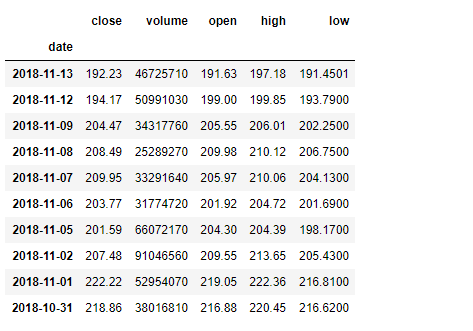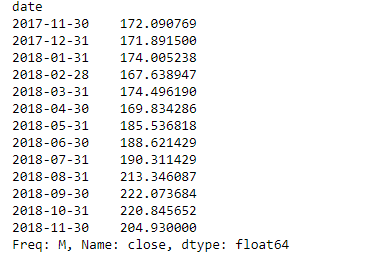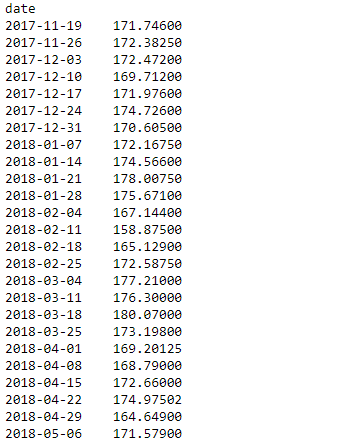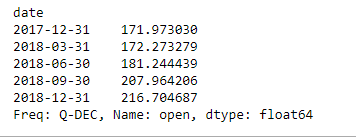# Python | Pandas dataframe.resample()

• Difficulty Level : Basic
• Last Updated : 22 Oct, 2019

Python is a great language for doing data analysis, primarily because of the fantastic ecosystem of data-centric python packages. Pandas is one of those packages and makes importing and analyzing data much easier.

Pandas` dataframe.resample()` function is primarily used for time series data.
A time series is a series of data points indexed (or listed or graphed) in time order. Most commonly, a time series is a sequence taken at successive equally spaced points in time. It is a Convenience method for frequency conversion and resampling of time series. Object must have a datetime-like index (DatetimeIndex, PeriodIndex, or TimedeltaIndex), or pass datetime-like values to the on or level keyword.

Attention geek! Strengthen your foundations with the Python Programming Foundation Course and learn the basics.

To begin with, your interview preparations Enhance your Data Structures concepts with the Python DS Course. And to begin with your Machine Learning Journey, join the Machine Learning - Basic Level Course

Syntax : DataFrame.resample(rule, how=None, axis=0, fill_method=None, closed=None, label=None, convention=’start’, kind=None, loffset=None, limit=None, base=0, on=None, level=None)

Parameters :
rule : the offset string or object representing target conversion
axis : int, optional, default 0
closed : {‘right’, ‘left’}
label : {‘right’, ‘left’}
convention : For PeriodIndex only, controls whether to use the start or end of rule
loffset : Adjust the resampled time labels
base : For frequencies that evenly subdivide 1 day, the “origin” of the aggregated intervals. For example, for ‘5min’ frequency, base could range from 0 through 4. Defaults to 0.
on : For a DataFrame, column to use instead of index for resampling. Column must be datetime-like.
level : For a MultiIndex, level (name or number) to use for resampling. Level must be datetime-like.

Resampling generates a unique sampling distribution on the basis of the actual data. We can apply various frequency to resample our time series data. This is a very important technique in the field of analytics.
Most commonly used time series frequency are –
W : weekly frequency
M : month end frequency
SM : semi-month end frequency (15th and end of month)
Q : quarter end frequency

There are many other types of time series frequency available. Let’s see how to apply these time series frequency on data and resample it.

This is a stock price data of Apple for a duration of 1 year from (13-11-17) to (13-11-18)

Example #1: Resampling the data on monthly frequency

 `# importing pandas as pd``import` `pandas as pd`` ` `# By default the "date" column was in string format,``# we need to convert it into date-time format`` ` `# parse_dates =["date"], converts the "date" ``# column to date-time format. We know that ``# resampling works with time-series data only``# so convert "date" column to index`` ` `# index_col ="date", makes "date" column, the index of the data frame``df ``=` `pd.read_csv(``"apple.csv"``, parse_dates ``=``[``"date"``], index_col ``=``"date"``)`` ` `# Printing the first 10 rows of dataframe``df[:``10``]``# Resampling the time series data based on months``# we apply it on stock close price``# 'M' indicates month``monthly_resampled_data ``=` `df.close.resample(``'M'``).mean()`` ` `# the above command will find the mean closing price``# of each month for a duration of 12 months.``monthly_resampled_data`

Output :Example #2: Resampling the data on weekly frequency

 `# importing pandas as pd``import` `pandas as pd`` ` `# We know that resampling works with time-series data``# only so convert "date" column to index``# index_col ="date", makes "date" column.`` ` `df ``=` `pd.read_csv(``"apple.csv"``, parse_dates ``=``[``"date"``], index_col ``=``"date"``)`` ` `# Resampling the time series data based on weekly frequency``# we apply it on stock open price 'W' indicates week``weekly_resampled_data ``=` `df.``open``.resample(``'W'``).mean()`` ` `# find the mean opening price of each week ``# for each week over a period of 1 year.``weekly_resampled_data`

Output :Example #3: Resampling the data on Quarterly frequency

 `# importing pandas as pd``import` `pandas as pd`` ` `# We know that resampling works with time-series``#  data only so convert our "date" column to index``# index_col ="date", makes "date" column``df ``=` `pd.read_csv(``"apple.csv"``, parse_dates ``=``[``"date"``], index_col ``=``"date"``)`` ` `# Resampling the time series data``#  based on Quarterly frequency``# 'Q' indicates quarter`` ` `Quarterly_resampled_data ``=` `df.``open``.resample(``'Q'``).mean()`` ` `# mean opening price of each quarter``# over a period of 1 year.``Quarterly_resampled_data`

Output :My Personal Notes arrow_drop_up